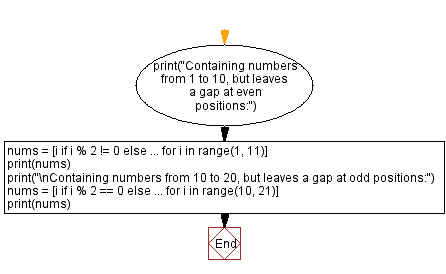﻿ Python Program: Creating lists with gaps using ellipsis

# Python Program: Creating lists with gaps using ellipsis

## Python ellipsis (...) Data Type: Exercise-4 with Solution

Write a Python program that creates a list containing numbers from 1 to 10, but leaves a gap at even positions using ellipsis.

Sample Solution:

Code:

``````print("Containing numbers from 1 to 10, but leaves a gap at even positions:")
nums = [i if i % 2 != 0 else ... for i in range(1, 11)]
print(nums)
print("\nContaining numbers from 10 to 20, but leaves a gap at odd positions:")
nums = [i if i % 2 == 0 else ... for i in range(10, 21)]
print(nums)
``````

Output:

```Containing numbers from 1 to 10, but leaves a gap at even positions:
[1, Ellipsis, 3, Ellipsis, 5, Ellipsis, 7, Ellipsis, 9, Ellipsis]

Containing numbers from 10 to 20, but leaves a gap at odd positions:
[10, Ellipsis, 12, Ellipsis, 14, Ellipsis, 16, Ellipsis, 18, Ellipsis, 20]
```

In the code above, we use a list comprehension to iterate through numbers from 1 to 10. If the number is odd, it's included in the list as is. However, if it's even, we use the ellipsis (...) to represent the gap.

Next, we use a list comprehension to iterate through numbers from 10 to 20. If the number is even, it's included in the list as is. However, if it's odd, we use the ellipsis (...) to represent the gap.

Flowchart:What is the difficulty level of this exercise?

Test your Programming skills with w3resource's quiz.

﻿

## Python: Tips of the Day

Memory Footprint Of An Object:

```import sys
```60